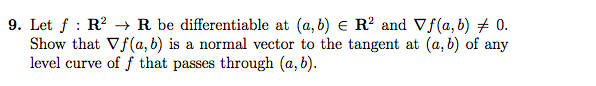# (Solved): Can Someone Please Give Me An Easy Proof, Just By Using Things You Learn In Calculus 3. Thank U. ...Can someone please give me an easy proof, just by using things you learn in calculus 3. thank u.

9. Let f : RÂ² + R be differentiable at (a,b) e R2 and Vf(a,b) + 0. Show that f(a,b) is a normal vector to the tangent at (a,b) of any level curve off that passes through (a,b).

We have an Answer from Expert